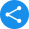# NCERT Book Class 12 Physics PDF

## CBSE Class 12 NCERT Books for Physics PDF - Free Download

Students who opted to study Science at 10+2 level; physics is one major subject which they will have in their curriculum. However, students often get confused about how to start with the chapters because of the vast syllabus. In such a scenario, you can start your lesson by downloading NCERT Class 12 Physics Book PDF.

 Class 12th Board CBSE Book NCERT Subject Physics Medium English / Hindi Study Materials Free Study Materials Available PDF Solutions Available in PDF Part 1 NCERT Physics Book Class 12 Part 1 PDF Download Part 2 NCERT Physics Book Class 12 Part 2 PDF Download

We have curated solutions for the books that you follow which you can access from the below links.

### NCERT Solutions for Class 12

Q 1: What is the syllabus of CBSE Class 12 Physics?

Ans: Altogether there are 14 chapters in Class 12 Physics. Below is the list of chapters:

• Unit -1: Electrostatics

1. Chapter 1: Electric Charges and Field

2. Chapter 2: Electrostatic Potential and capacitance.

• Unit - 2: Current Electricity

1. Chapter 3: Current Electricity

• Unit - 3: Magnetic Effects of Magnetism and Current

1. Chapter 4: Moving Charges and Magnetism

2. Chapter 5: Magnetism and Matter

• Unit - 4: Electromagnetic Induction and Alternating Currents

1. Chapter 6: Electromagnetic Induction

2. Chapter 7: Alternating Current

• Unit - 5: Electromagnetic Waves

1. Chapter 8: Electromagnetic Waves

• Unit - 6: Optics

1. Chapter 9: Ray optics and optical instruments

2. Chapter 10: Wave optics

• Unit - 7: Dual Nature of Matter and Radiation

1. Chapter 11: Dual nature of radiation and matter

• Unit - 8: Atoms and Nuclei

1. Chapter 12: Atoms

2. Chapter 13: Nuclei

• Unit - 9: Electronic Devices

1. Chapter 14: Semiconductor of electronics: devices, simple circuits and materials

2. Chapter 15: Communication system

Q 2: How to attempt a physics paper on the mainboard exam?

Ans: Firstly, make a note of all the formulas and revise them often to remember them by heart. Derivations play a key role in Physics. Learning the derivations of the Formulas will help you solve the questions on board with ease. Questions in the exam will be asked in a deviant way. To understand them you need to be very much perfect with the derivations of the formulas.

Always focus mainly on the topics with a high weightage of marks. Your main attention should be on concepts like a Sound wave, Wave Optics, Modern Physics, Alternating current. Thermodynamics is a subject which is common in both the subjects of Physics and Chemistry. This is also one of the most important topics in Physics. Thorough practice with all the concepts is a must.

Q 3: What will I learn in Fluid mechanics chapter?

Ans: In Fluid Mechanics chapter, you will be taught about Bernoulli’s Equation, Archimedes’ Principle, Pascal’s Law, Applications of Bernoulli’s Equation, the flow of fluids, incompressible and nonviscous fluid, atmospheric pressure and barometer and equation of continuity. The concept Some Mechanical Properties of Matter gives you a brief on Stokes’ Law, Hooke’s Law and the Modulus of Elasticity, Stokes’ Method, Poiseuille’s Equation, surface tension, the molecular structure of a material, critical velocity and Reynolds Number.

Q 4: What are the main concepts which I learn in the chapter Motion in 1 dimension?

Ans: Motion in 1 Dimension is the most important chapter of class 12 Physics. The chapter introduces you to the introduction to mechanics and kinematics, basic definitions, motion in one, two and three dimensions, uniformly accelerated motion, non - accelerated motion, graphs and relative motion uniformly.

This main focus of the chapter is on the moment of an object and how it changes its position with proportionate to the surrounding along with the time. Further, you will also learn about the types of linear motion like uniform linear motion and non-uniform linear motion, which includes vector quantities. These quantities are explained with linear momentum, acceleration, displacement, momentum, angular velocity, force, electric field and polarization.

Share this with your friendsShare